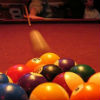2.003SC | Fall 2011 | Undergraduate

# Engineering Dynamics

## Newton's Laws, Vectors, and Reference Frames

« Previous | Next »

### Session OverviewIn week 1, we introduce Newton’s three laws of motion. We use them to write an equation of motion. Equations of motion require the evaluation of displacements, velocities, and accelerations. The study of motion is called kinematics. In this course, we use vectors to describe the motion of rigid bodies. The equations for vector displacements, velocities, and accelerations are developed in the first week. Billiards is a game that requires a familiarity with Newton’s laws. Image courtesy of fictures on Flickr.

### Assignments

#### Problems and Concept Questions

Each problem set has concept questions paired with most problems. Answers to the concept questions are handed in before the overall problem set is due. You should therefore answer the concept questions in each problem set while watching the video lectures for the week. Then, use the Concept Question Answer Key (below in the Check Yourself section) to check your work before continuing to work on the problem set.

Please note that if your answer to the concept question is incorrect, it is likely that you may start down an unproductive pathway to a solution. Try to fully understand the answer to the concept question before you begin the regular problem.

#### Handouts

• [Hibbeler] sections 12.1-12.6, 13.1-13.6, 16.1-16.6, 16.8.

### Videos

#### Lecture Videos

• Watch Notation Systems, a supplementary video which describes the two notation systems used in this course and how they interrelate.

### Check Yourself

#### Concept Question Answer Key and Problem Set Solutions

The solutions are presented in two files, one with the answers to the concept questions, and one with solutions and in-depth explanations for the problems. Work the problems on your own and check your answers when you’re done.

Looking for something specific in this course? The Resource Index compiles links to most course resources in a single page.

« Previous | Next »

## Course Info

Fall 2011
##### Learning Resource Types
Lecture Videos
Problem Sets with Solutions
Exams with Solutions
Recitation Videos
Lecture Notes